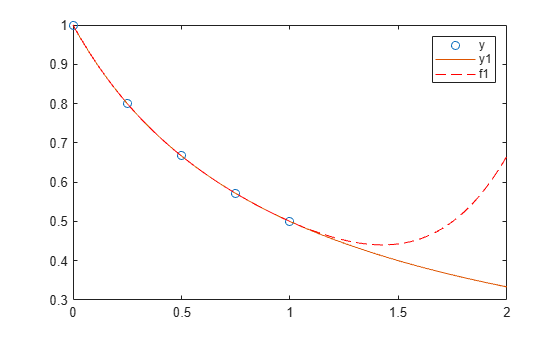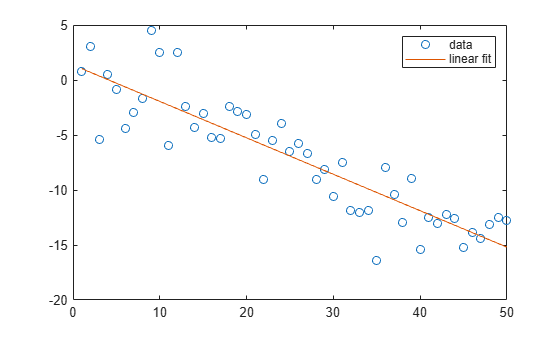polyfit

Polynomial curve fitting

Description

example

p = polyfit(x,y,n) returns the coefficients for a polynomial p(x) of degree n that is a best fit (in a least-squares sense) for the data in y. The coefficients in p are in descending powers, and the length of p is n+1

$p\left(x\right)={p}_{1}{x}^{n}+{p}_{2}{x}^{n-1}+...+{p}_{n}x+{p}_{n+1}.$

[p,S] = polyfit(x,y,n) also returns a structure S that can be used as an input to polyval to obtain error estimates.

example

[p,S,mu] = polyfit(x,y,n) also returns mu, which is a two-element vector with centering and scaling values. mu(1) is mean(x), and mu(2) is std(x). Using these values, polyfit centers x at zero and scales it to have unit standard deviation,

$\stackrel{^}{x}=\frac{x-\overline{x}}{{\sigma }_{x}}\text{\hspace{0.17em}}.$

This centering and scaling transformation improves the numerical properties of both the polynomial and the fitting algorithm.

Examples

collapse all

Generate 10 points equally spaced along a sine curve in the interval [0,4*pi].

x = linspace(0,4*pi,10);
y = sin(x);

Use polyfit to fit a 7th-degree polynomial to the points.

p = polyfit(x,y,7);

Evaluate the polynomial on a finer grid and plot the results.

x1 = linspace(0,4*pi);
y1 = polyval(p,x1);
figure
plot(x,y,'o')
hold on
plot(x1,y1)
hold offCreate a vector of 5 equally spaced points in the interval [0,1], and evaluate $y\left(x\right)=\left(1+x{\right)}^{-1}$ at those points.

x = linspace(0,1,5);
y = 1./(1+x);

Fit a polynomial of degree 4 to the 5 points. In general, for n points, you can fit a polynomial of degree n-1 to exactly pass through the points.

p = polyfit(x,y,4);

Evaluate the original function and the polynomial fit on a finer grid of points between 0 and 2.

x1 = linspace(0,2);
y1 = 1./(1+x1);
f1 = polyval(p,x1);

Plot the function values and the polynomial fit in the wider interval [0,2], with the points used to obtain the polynomial fit highlighted as circles. The polynomial fit is good in the original [0,1] interval, but quickly diverges from the fitted function outside of that interval.

figure
plot(x,y,'o')
hold on
plot(x1,y1)
plot(x1,f1,'r--')
legend('y','y1','f1')First generate a vector of x points, equally spaced in the interval [0,2.5], and then evaluate erf(x) at those points.

x = (0:0.1:2.5)';
y = erf(x);

Determine the coefficients of the approximating polynomial of degree 6.

p = polyfit(x,y,6)
p = 1×7

0.0084   -0.0983    0.4217   -0.7435    0.1471    1.1064    0.0004

To see how good the fit is, evaluate the polynomial at the data points and generate a table showing the data, fit, and error.

f = polyval(p,x);
T = table(x,y,f,y-f,'VariableNames',{'X','Y','Fit','FitError'})
T=26×4 table
X        Y          Fit         FitError
___    _______    __________    ___________

0          0    0.00044117    -0.00044117
0.1    0.11246       0.11185     0.00060836
0.2     0.2227       0.22231     0.00039189
0.3    0.32863       0.32872    -9.7429e-05
0.4    0.42839        0.4288    -0.00040661
0.5     0.5205       0.52093    -0.00042568
0.6    0.60386       0.60408    -0.00022824
0.7     0.6778       0.67775     4.6383e-05
0.8     0.7421       0.74183     0.00026992
0.9    0.79691       0.79654     0.00036515
1     0.8427       0.84238      0.0003164
1.1    0.88021       0.88005     0.00015948
1.2    0.91031       0.91035    -3.9919e-05
1.3    0.93401       0.93422      -0.000211
1.4    0.95229       0.95258    -0.00029933
1.5    0.96611       0.96639    -0.00028097
⋮

In this interval, the interpolated values and the actual values agree fairly closely. Create a plot to show how outside this interval, the extrapolated values quickly diverge from the actual data.

x1 = (0:0.1:5)';
y1 = erf(x1);
f1 = polyval(p,x1);
figure
plot(x,y,'o')
hold on
plot(x1,y1,'-')
plot(x1,f1,'r--')
axis([0  5  0  2])
hold offCreate a table of population data for the years 1750 - 2000 and plot the data points.

year = (1750:25:2000)';
pop = 1e6*[791 856 978 1050 1262 1544 1650 2532 6122 8170 11560]';
T = table(year, pop)
T=11×2 table
year       pop
____    _________

1750     7.91e+08
1775     8.56e+08
1800     9.78e+08
1825     1.05e+09
1850    1.262e+09
1875    1.544e+09
1900     1.65e+09
1925    2.532e+09
1950    6.122e+09
1975     8.17e+09
2000    1.156e+10

plot(year,pop,'o')Use polyfit with three outputs to fit a 5th-degree polynomial using centering and scaling, which improves the numerical properties of the problem. polyfit centers the data in year at 0 and scales it to have a standard deviation of 1, which avoids an ill-conditioned Vandermonde matrix in the fit calculation.

[p,~,mu] = polyfit(T.year, T.pop, 5);

Use polyval with four inputs to evaluate p with the scaled years, (year-mu(1))/mu(2). Plot the results against the original years.

f = polyval(p,year,[],mu);
hold on
plot(year,f)
hold offFit a simple linear regression model to a set of discrete 2-D data points.

Create a few vectors of sample data points (x,y). Fit a first degree polynomial to the data.

x = 1:50;
y = -0.3*x + 2*randn(1,50);
p = polyfit(x,y,1);

Evaluate the fitted polynomial p at the points in x. Plot the resulting linear regression model with the data.

f = polyval(p,x);
plot(x,y,'o',x,f,'-')
legend('data','linear fit')Fit a linear model to a set of data points and plot the results, including an estimate of a 95% prediction interval.

Create a few vectors of sample data points (x,y). Use polyfit to fit a first degree polynomial to the data. Specify two outputs to return the coefficients for the linear fit as well as the error estimation structure.

x = 1:100;
y = -0.3*x + 2*randn(1,100);
[p,S] = polyfit(x,y,1);

Evaluate the first-degree polynomial fit in p at the points in x. Specify the error estimation structure as the third input so that polyval calculates an estimate of the standard error. The standard error estimate is returned in delta.

[y_fit,delta] = polyval(p,x,S);

Plot the original data, linear fit, and 95% prediction interval $\mathit{y}±2\Delta$.

plot(x,y,'bo')
hold on
plot(x,y_fit,'r-')
plot(x,y_fit+2*delta,'m--',x,y_fit-2*delta,'m--')
title('Linear Fit of Data with 95% Prediction Interval')
legend('Data','Linear Fit','95% Prediction Interval')Input Arguments

collapse all

Query points, specified as a vector. The points in x correspond to the fitted function values contained in y. If x is not a vector, then polyfit converts it into a column vector x(:).

Warning messages result when x has repeated (or nearly repeated) points or if x might need centering and scaling.

Data Types: single | double
Complex Number Support: Yes

Fitted values at query points, specified as a vector. The values in y correspond to the query points contained in x. If y is not a vector, then polyfit converts it into a column vector y(:).

Data Types: single | double
Complex Number Support: Yes

Degree of polynomial fit, specified as a positive integer scalar. n specifies the polynomial power of the left-most coefficient in p.

Output Arguments

collapse all

Least-squares fit polynomial coefficients, returned as a vector. p has length n+1 and contains the polynomial coefficients in descending powers, with the highest power being n. If either x or y contain NaN values and n < length(x), then all elements in p are NaN.

Use polyval to evaluate p at query points.

Error estimation structure. This optional output structure is primarily used as an input to the polyval function to obtain error estimates. S contains the following fields:

FieldDescription
RTriangular R factor (possibly permuted) from a QR decomposition of the Vandermonde matrix of x
dfDegrees of freedom
normrNorm of the residuals

If the data in y is random, then an estimate of the covariance matrix of p is (Rinv*Rinv')*normr^2/df, where Rinv is the inverse of R.

If the errors in the data in y are independent and normal with constant variance, then [y,delta] = polyval(...) produces error bounds that contain at least 50% of the predictions. That is, y ± delta contains at least 50% of the predictions of future observations at x.

Centering and scaling values, returned as a two-element vector. mu(1) is mean(x), and mu(2) is std(x). These values center the query points in x at zero with unit standard deviation.

Use mu as the fourth input to polyval to evaluate p at the scaled points, (x - mu(1))/mu(2).

Limitations

• In problems with many points, increasing the degree of the polynomial fit using polyfit does not always result in a better fit. High-order polynomials can be oscillatory between the data points, leading to a poorer fit to the data. In those cases, you might use a low-order polynomial fit (which tends to be smoother between points) or a different technique, depending on the problem.

• Polynomials are unbounded, oscillatory functions by nature. Therefore, they are not well-suited to extrapolating bounded data or monotonic (increasing or decreasing) data.

Algorithms

polyfit uses x to form Vandermonde matrix V with n+1 columns and m = length(x) rows, resulting in the linear system

$\left(\begin{array}{cccc}{x}_{1}^{n}& {x}_{1}^{n-1}& \cdots & 1\\ {x}_{2}^{n}& {x}_{2}^{n-1}& \cdots & 1\\ ⋮& ⋮& \ddots & ⋮\\ {x}_{m}^{n}& {x}_{m}^{n-1}& \cdots & 1\end{array}\right)\left(\begin{array}{c}{p}_{1}\\ {p}_{2}\\ ⋮\\ {p}_{n+1}\end{array}\right)=\left(\begin{array}{c}{y}_{1}\\ {y}_{2}\\ ⋮\\ {y}_{m}\end{array}\right)\text{\hspace{0.17em}}\text{\hspace{0.17em}},$

which polyfit solves with p = V\y. Since the columns in the Vandermonde matrix are powers of the vector x, the condition number of V is often large for high-order fits, resulting in a singular coefficient matrix. In those cases centering and scaling can improve the numerical properties of the system to produce a more reliable fit.